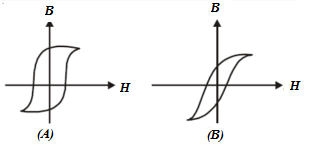# Magnetism MCQs for NEET

NEET  Physics is the scoring paper in the medical entrance examination. Here, you will discover the NEET Physics MCQ Questions for all Concepts as per the latest syllabus. Practice more on a regular basis with these NEET Physics objective questions on air pollution and improve your subject knowledge & problem-solving skills along with time management. NEET Physics Magnetism Multiple Choice Questions make you feel confident in answering the question in the exam & increases your scores to high.

## MCQ on Magnetism

1. For which of the following is magnetic susceptibility negative?
(a) Paramagnetic and Ferromagnetic materials
(b) Paramagnetic Materials only
(c) Ferromagnetic Materials only
(d) Diamagnetic Materials

2. The Hysteresis loops for two magnetic materials A and B are given below:These materials are used to make magnets for transformer core, electric generators and electromagnet core. Which of the following is true about the given magnets?
(a) A for transformers and B for electric generators
(b) A for electric generators and transformers
(c) B for electromagnets and generators
(d) A for electromagnets and B for electric generators

Answer: (c) B for electromagnets and generators

3. Which of the following is the unit of magnetic flux density?
(a) Weber/meter2
(b) Tesla
(c) Newton/ampere-metre
(d) All of the above

4. The magnetism of a magnet is due to
(a) earth
(b) cosmic rays
(c) due to pressure of big magnet inside the earth
(d) spin motion of electrons

Answer: (d) spin motion of electrons

5. The magnetic field at a point x on the axis of a mall bar magnet is equal to the field at a point y on the equator of the same magnet. The ratio of the distances of x and y from the centre of the magnet is
(a) 2-1/3
(b) 21/3
(c) 23
(d) 2-3

6. Which of the following materials is the most suitable for making a permanent magnet?
(a) Soft Iron
(b) Nickel
(c) Copper
(d) Steel

7. A sensitive magnetic field instrument can be effectively shielded from the external magnetic field by placing it inside which of the following materials?
(a) Plastic Material
(b) Teak Wood
(c) Soft Iron of high permeability
(d) A metal of high conductivity

Answer: (c) Soft Iron of high permeability

8. A long magnet is cut into two parts such that the ratio of their lengths is 2:1. What is the ratio pole strength of both the section?
(a) 1:2
(b) 2:1
(c) 4:1
(d) Equal

9. Which of the following statements is true about magnetic field intensity?
(a) Magnetic field intensity is the number of lines of force crossing per unit volume.
(b) Magnetic field intensity is the number of lines of force crossing per unit area.
(c) Magnetic field intensity is the magnetic induction force acting on a unit magnetic pole.
(d) Magnetic field intensity is the magnetic moment per unit volume.

Answer: (c) Magnetic field intensity is the magnetic induction force acting on a unit magnetic pole

10. What happens to the magnetic needle kept in a non-uniform magnetic field?
(a) It experiences force but not torque
(b) It experiences torque but not force
(c) It experiences both force and torque
(d) It neither experiences force nor torque

Answer: (a) It experiences force but not torque

11. What is the torque acting on the bar magnet of moment M which is placed in a uniform field H making an angle of 300 with the field?
(a) MH
(b) MH/2
(c) MH/3
(d) MH/4

12. What happens to the magnetic moment if a hole is made at the centre of a bar magnet?
(a) Decreases
(b) Increases
(c) Not a change
(d) None of the above

13. Which of the following statements is true about magnetic lines of force?
(a) Magnetic lines of force are always closed.
(b) Magnetic lines of force always intersect each other.
(c) Magnetic lines of force tend to crowd far away from the poles of the magnet
(d) Magnetic lines of force do not pass through the vacuum.

Answer: (a) Magnetic lines of force are always closed.

14. What is the work done by the magnet of moment M if it is rotated through 3600 in magnetic field H?
(a) 2MH
(b) MH
(c) 2πMH
(d) Zero